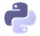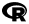# Data Science Skill Paths

Each Skill Path is a curated sequence of lessons, teaching you everything you need to know on the most popular data topics.

### SQL Fundamentals

Learn how to extract data from databases using SQL.

• How to use SQL to explore and extract data
• How to use SQL to organize data
• How to write SQL queries to find summary statistics
• How to query across multiple tables

### Machine Learning Introduction with Python

• The basics of machine learning, including avoiding common pitfalls and evaluating model performance
• Common techniques like k-nearest neighbours, k-means clustering, and decision trees
• Foundational mathematics for machine learning, including calculus and linear algebra

### Machine Learning Intermediate with Python

• How to work with neural networks
• The basics of building a machine learning project from start to finish
• How to build a machine learning model and make your first Kaggle submission
• How to select the best algorithm and tune your model for best performance

### Data Visualization with R

Learn how to use R for data visualization.

• How to visualize changes overtime with line graphs
• How to use histograms to understand data distributions
• How to compare groups using bar charts and box plots
• How to understand relationships between variables using scatter plots### Data Analysis and Visualization with Python

Learn how Python and Pandas simplify data analysis and visualization.

• How to use Python libraries Pandas and NumPy for data analysis
• How to use the most common data visualization techniques to explore data
• How to use data visualization to convey insights and tell a story
• Techniques for manipulating and cleaning data### APIs and Web Scraping with R

Learn how to acquire data from APIs and the web with R.

• How to query external data sources with an API using R
• The basics of scraping data from the web### Python Basics for Data Analysis

Introduce yourself to the python programming language.

• Fundamentals of programming in Python
• How to use Jupyter notebooks
• How to work with text, date, and time data
• Basics of object-oriented programming in Python### Probability and Statistics with Python

Learn probability and statistics for more robust data analysis.

• Basics of statistical analysis, including sampling, working with variables, and understanding frequency distribution tables
• How to summarize a distribution's measures of central tendency and variability
• The fundamentals of probability and how to use them for analysis
• How to create and test hypotheses using significance testing

### R Basics for Data Analysis

Introduce yourself to the R programming language.

• The fundamentals of programming in R
• How to use control and flow iteration
• How to work with and create your own functions
• The basics of working with strings and dates

### APIs and Web Scraping with Python

Learn how to acquire data from APIs and the web with Python.

• How to query external data sources with an API using Python
• The basics of scraping data from the web

### Probability and Statistics with R

Learn probability and statistics for more robust data analysis.

• The basics of statistical analysis, including sampling, working with variables, and understanding frequency distribution tables
• How to summarize a distribution's measures of central tendency and variability
• The fundamentals of probability and how to use them for analysis
• How to create and test hypotheses using significance testing

### Not finding what you're looking for?

We're always adding new content and would love to hear from you.

` (function() { var qs,js,q,s,d=document, gi=d.getElementById, ce=d.createElement, gt=d.getElementsByTagName, id="typef_orm_share", b="https://embed.typeform.com/"; if(!gi.call(d,id)){ js=ce.call(d,"script"); js.id=id; js.src=b+"embed.js"; q=gt.call(d,"script"); q.parentNode.insertBefore(js,q) } })() `
Looking for our Career Paths? See our full course catalog →